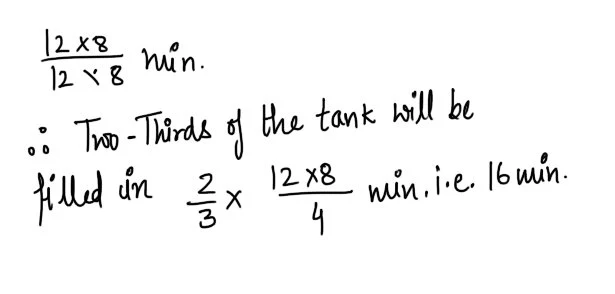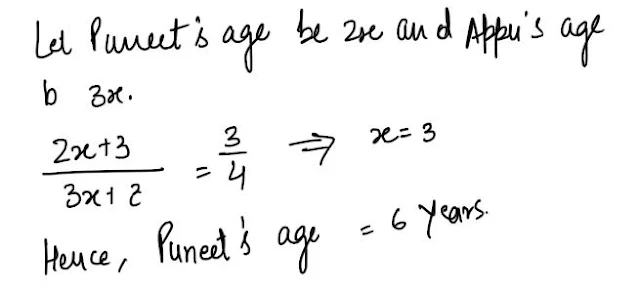Gurpurab Offer - Use Code GURPURAB2023

# Mixed Aptitude Quiz - 11 (For SBI Clerical 2016)#### Ques 1.

The L.C.M. of two numbers x and y is a . The H.C.F. of x and Y will be.
(a) ax/y
(b) xy/a
(c) a/xy
(d) xy
Ans 1.#### Ques 2.

Find the unit's digit in the product :
(a) 274 x 318 x 577 x 313
(a) 3
(b) 2
(c) 4
(d) 5
Ans 2. Required unit's  digit  is the unit's digit in the product  4 x 8 x 7 x 3 = 2 .

#### Ques 3.

If I had walked 1 km/hr faster, I would have taken 10 minutes less to walk 2 km. Find the speed of my walking
(a) 4 km/hr
(b) 5 km/hr
(c) 3 km/hr
(d) None of these
Ans 3.#### Ques 4.

Pipe A can fill a tank in 8 min and pipe B can empty it in 12 min.In what time will two- thirds of the tank be filled if both are opened together ?
(a) 15 min
(b) 16 min
(c) 18 min
(d) 5 min
Ans 4. Time taken by the  tank to get filled up when pipe A and B both are opened =#### Ques 5.

The sum of squares of 2 numbers is 801. If one of the numbers is 15, find the other.
(a) 24,4
(b) 24.04
(c) 24
(d) None of these
Ans 5.#### Ques 6.

Amit bought 15 kg sugar at the rate of Rs 14.50 per kg and 10 kg sugar at the rate of Rs 13 per kg. He mixed both the qualities and sold the mixture at the rate of Rs 15 per kg .How much did he gain ( in Rs )?
(a) 1.10
(b) 16.5
(c) 11
(d) 27.50
Ans 6. Amit's gain = Rs{ 15 x ( 15 + 10 ) -
( 14.50 x 15 ) - ( 13 x 10) }
= Rs { 375 - 217.5 -130}
=Rs 27.50

#### Ques 7.

3 men can do a work in 6 days. After 2 Days 3 more than joined thyem .How many will they take to complete the remaining work ?
(a) 4
(b) 2
(c) 3
(d) 5
Ans 7.#### Ques 8.

Sanjeev and Rajesh are partners in a business Sanjeev invests Rs 5000 for 5 months and Rajesh invests Rs 6000 for 6 months .If the profit is Rs 610, then Rajesh's share in the profit is
(a) Rs 250
(b) Rs 360
(c) Rs 520
(d) Rs 610
Ans 8.#### Ques 9.

The difference between compound interest and simple interest on a certain sum of money in 2 years at 4% p.a. is Rs 50.The principal is
(a) Rs 20400
(b) Rs 31250
(c) Rs 25000
(d) None of these
Ans 9.#### Ques 10.

The Ratio of the present ages of Puneet and Appu is 2 : 3 After 3 years the ratio will be 3 :4 .What is the present age of puneet ?
(a) 6 years
(b) 9 years
(c) 15 years
(d) None of these
Ans 10.